# Slope of a Line

The slope $m$ of a line passing through two points $\left({x}_{1},{y}_{1}\right)$ and $\left({x}_{2},{y}_{2}\right)$ is:

$m=\frac{{y}_{2}\text{\hspace{0.17em}}-\text{\hspace{0.17em}}{y}_{1}}{{x}_{2}\text{\hspace{0.17em}}-\text{\hspace{0.17em}}{x}_{1}}$

If the graph of a line rises from left to right, the slope is positive.  If the graph of the line falls from left to right the slope is negative.

Example:

Find the slope of the line passing through the points $\left(-3,17\right)$ and $\left(4,3\right)$.

Substituting ${x}_{1}=-3,{y}_{1}=17,{x}_{2}=4,\text{\hspace{0.17em}}\text{\hspace{0.17em}}\text{and}\text{\hspace{0.17em}}\text{\hspace{0.17em}}{y}_{2}=3$, we get:

$m=\frac{3\text{\hspace{0.17em}}-\text{\hspace{0.17em}}17}{4\text{\hspace{0.17em}}-\text{\hspace{0.17em}}\left(-3\right)}=\frac{-14}{7}=-2$

So the slope is $-2$.

## You can follow the steps below to find the slope of any line.

$1$) Pick any two points on the line. (Pick points with integer coordinates to make your life easier.)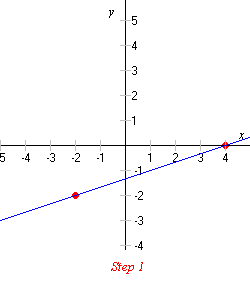$2$) Draw a vertical line going down from the higher point.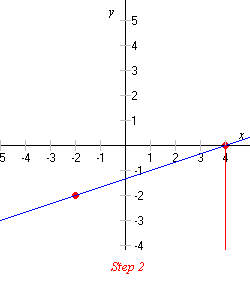$3$) Draw a horizontal line from the other point so that it meets the vertical line.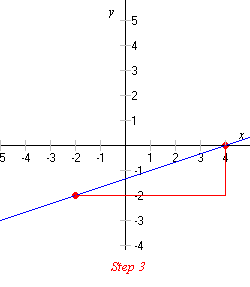$4$) You now have a right triangle, called a slope triangle. Find the lengths of the vertical and the horizontal legs.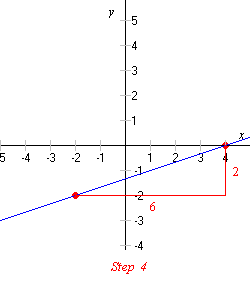$5$) Divide the length of the vertical leg (the “rise”) by the length of the horizontal leg (the “run”). This quotient is the slope of the line.

$\frac{\text{rise}}{\text{run}}=\frac{2}{6}=\frac{1}{3}$

So the slope of the line in this example is $\frac{1}{3}$.

If the right angle is on the left side of the triangle, the slope is negative.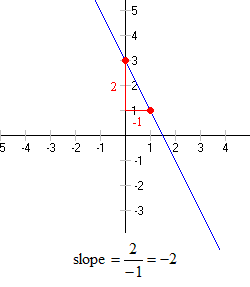## Horizontal and Vertical Lines

Horizontal lines have slope zero, since the “rise” is zero.

Vertical lines have undefined slope, since the “run” is zero, and division by zero is not allowed.